Computational & Technology Resources
an online resource for computational,
engineering & technology publications
Civil-Comp Proceedings
ISSN 1759-3433
CCP: 79
PROCEEDINGS OF THE SEVENTH INTERNATIONAL CONFERENCE ON COMPUTATIONAL STRUCTURES TECHNOLOGY
Edited by: B.H.V. Topping and C.A. Mota Soares
Paper 118

Numerical Integration of Interpolation and Test Functions on any Convex Polyhedrons

Institute of Computer Science in Civil Engineering, University of Hannover, Germany

Full Bibliographic Reference for this paper
T. Fröbel, P. Milbradt, "Numerical Integration of Interpolation and Test Functions on any Convex Polyhedrons", in B.H.V. Topping, C.A. Mota Soares, (Editors), "Proceedings of the Seventh International Conference on Computational Structures Technology", Civil-Comp Press, Stirlingshire, UK, Paper 118, 2004. doi:10.4203/ccp.79.118
Keywords: convex polyhedrons, natural element coordinates, quadrature formulas.

Summary
The finite element method is used to simulate processes in science, nature and engineering to solve descriptive partial differential equations. As geometrical basis of finite element method triangles and quadrangles in the 2-dimensional space are used as well as tetrahedrons and cubes in the 3-dimensional space. In this paper the use of any convex polyhedrons as finite elements is introduced.

The formulation of a local coordinate system makes a uniform description of interpolation and test functions possible. In this paper natural element coordinates for any convex polyhedrons  will be introduced. It has been shown that natural element coordinates could be conceived as generalized barycentric coordinates. Every edge of the convex polyhedron can be matched a local coordinate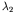.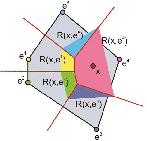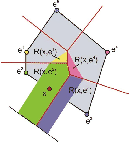To assess the natural element coordinate for a point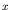the VORONOI-decomposition of first order and the VORONOI-decomposition of second order are drawn according the point.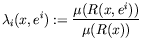(48)

The natural element coordinate can be compute by the ratio of the LEBESGUE-measure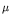of the VORONOI-region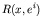and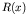. The use of natural element coordinates enables a geometry independence formulation of interpolation and test functions .

In the case of finite element approximation  the differentiation and integration of these interpolations and test functions are necessary.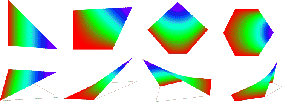In this paper especially the properties of the natural element coordinates whose differential- and integration ability are brought to attention. The necessary methods and their numerical implementation are introduced and advantages and disadvantages are described.

Finally, applications from the range of interpolation of data and approximations of solutions of partial differential equations in field of mechanics are presented.

References
1
P. Milbradt, "Algorithmische Geometrie in der Bauinformatik", Habilitationsschrift, Universität Hannover, 2001
2
J. Schierbaum, A. Schwöppe, "Ansatzfunktionen höherer Ordnung für Finite konvexe Zellen", Forum Bauinformatik 2003
3
P. Milbradt, A. Schwöppe, "Finite Element Approximation auf der Basis geometrischer Zellen", IKM, Weimar 2003

purchase the full-text of this paper (price £20)